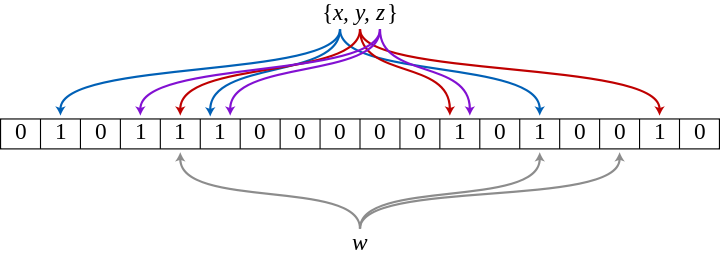# 大量数据去重：Bitmap和布隆过滤器(Bloom Filter)

5TB的硬盘上放满了数据，请写一个算法将这些数据进行排重。如果这些数据是一些32bit大小的数据该如何解决？如果是64bit的呢？

## Bitmap算法

public int[] removeDuplicates(int[] array) {
int index = 0;
int[] newArray = new int[array.length];
Map<Integer, Boolean> maps = new LinkedHashMap<Integer, Boolean>();
for(int num : array) {
if(!maps.contains(num)) {
newArray[index++] = num;
maps.put(num, true);
}
}

return newArray;
}


1. 集合中每个元素（示例中是int）有一个独享的空间
2. 找到一个到这个空间的映射方法

2 32 b i t = 2 29 B y t e = 512 M B 2^{32} bit = 2^{29} Byte = 512 MB

public static final int _1MB = 1024 * 1024;
//每个byte记录8bit信息,也就是8个数是否存在于数组中
public static byte[] flags = new byte[ 512 * _1MB ];

public static void main(String[] args) {
//待判重数据
int[] array = {255, 1024, 0, 65536, 255};

int index = 0;
for(int num : array) {
if(!getFlag(num)) {
//未出现的元素
array[index] = num;
index = index + 1;
//设置标志位
setFlag(num);
System.out.println("set " + num);
} else {
}
}
}

public static void setFlag(int num) {
//使用每个数的低三位作为byte内的映射
//例如: 255 = (11111111)
//低三位(也就是num & (0x07))为(111) = 7, 则byte的第7位为1, 表示255已存在
flags[num >> 3] |= 0x01 << (num & (0x07));
}

public static boolean getFlag(int num) {
return (flags[num >> 3] >> (num & (0x07)) & 0x01) == 0x01;
}


static BitSet seen = new BitSet((1 << 31) - 1);
public static void setFlag(int num) {
seen.set(num);
}
public static boolean getFlag(int num) {
return set.get(num);
}


## 布隆过滤器（Bloom Filter）

2 64 b i t = 2 61 B y t e = 2048 P B = 2 E B 2^{64} bit = 2^{61} Byte = 2048 PB = 2 EB

EB（Exabyte，艾字节）这个计算机科学中统计数据量的单位有多大，有兴趣的小伙伴可以查阅下资料。这个量级的Bitmap，已经不是人类硬件所能承担的了。我相信谁也不会想用集群去计算这么一个问题吧1？所以Bitmap的好处在于空间复杂度不随原始集合内元素的个数增加而增加，而它的坏处也源于这一点——空间复杂度随集合内最大元素增大而线性增大x , y , z x,y,z 经由哈希函数映射将各自在Bitmap中的3个位置置为1，当 w w 出现时，仅当3个标志位都为1时，才表示 w w 在集合中。图中所示的情况，布隆过滤器将判定 w w 不在集合中。

1. 1个散列函数时，接收一个元素时Bitmap中某一位置为0的概率为：
1 − 1 m 1-\dfrac {1}{m}
2. k k 个相互独立的散列函数，接收一个元素时Bitmap中某一位置为0的概率为：
( 1 − 1 m ) k ({1-\dfrac {1}{m}})^k
3. 假设原始集合中，所有元素都不相等（最严格的情况），将所有元素都输入布隆过滤器，此时某一位置仍为0的概率为：
( 1 − 1 m ) n k ({1-\dfrac {1}{m}})^{nk}
某一位置为1的概率为：
1 − ( 1 − 1 m ) n k 1-({1-\dfrac {1}{m}})^{nk}
4. 当我们对某个元素进行判重时，误判即这个元素对应的 k k 个标志位不全为1，但所有 k k 个标志位都被置为1，误判率 ε \varepsilon 为：
ε ≈ [ 1 − ( 1 − 1 m ) n k ] k \varepsilon \approx [1-({1-\dfrac {1}{m}})^{nk}]^k
这个误判率应当比实际值大，因为将判断正确的情况也算进去了。根据著名极限 lim ⁡ n → ∞ ( 1 + 1 n ) n = e \lim\limits_{n\to\infty}{(1+\dfrac {1}{n})^n} = e 可以得到：
ε ≈ [ 1 − e − n k m ] k \varepsilon \approx [1-e^{-\frac{nk}{m}}]^k
ε \varepsilon 得到最优解2，当且仅当：
k = m n ln ⁡ 2 ≈ 0.7 m n k=\frac{m}{n} \ln2\approx 0.7\frac{m}{n}
此时，误判率 ε \varepsilon 与数集大小和
ε ≈ ( 1 − e − ln ⁡ 2 ) l n 2 m n = 0. 5 l n 2 m n = 0. 5 k \varepsilon \approx (1 - e ^{-\ln2}) ^ {ln2\frac{m}{n}} = 0.5 ^ {ln2\frac{m}{n}} = 0.5^k

n = 5 T B 64 b i t = 5 × 2 40 B y t e 8 B y t e ≈ 2 39 n = \dfrac{5TB }{ 64bit} = \dfrac{5 \times 2 ^{40}Byte}{8Byte} \approx 2^{39}

## 附录

import com.google.common.hash.BloomFilter;
import java.util.HashSet;
import java.util.Random;

public class testBloomFilter {

static int sizeOfNumberSet = Integer.MAX_VALUE >> 4;

static Random generator = new Random();

public static void main(String[] args) {

int error = 0;
HashSet<Integer> hashSet = new HashSet<Integer>();
BloomFilter<Integer> filter = BloomFilter.create(Funnels.integerFunnel(), sizeOfNumberSet);

for(int i = 0; i < sizeOfNumberSet; i++) {
int number = generator.nextInt();
if(filter.mightContain(number) != hashSet.contains(number)) {
error++;
}
filter.put(number);
}

System.out.println("Error count: " + error + ", error rate = " + String.format("%f", (float)error/(float)sizeOfNumberSet));
}
}


/**
* Creates a {@link BloomFilter BloomFilter<T>} with the expected number of
* insertions and a default expected false positive probability of 3%.
*/
public static <T> BloomFilter<T> create(Funnel<T> funnel, int expectedInsertions /* n */) {
return create(funnel, expectedInsertions, 0.03); // FYI, for 3%, we always get 5 hash functions
}


enum BloomFilterStrategies implements BloomFilter.Strategy {
/**
* See "Less Hashing, Same Performance: Building a Better Bloom Filter" by Adam Kirsch and
* Michael Mitzenmacher. The paper argues that this trick doesn't significantly deteriorate the
* performance of a Bloom filter (yet only needs two 32bit hash functions).
*/
MURMUR128_MITZ_32() {
@Override public <T> boolean put(T object, Funnel<? super T> funnel,
int numHashFunctions, BitArray bits) {
long hash64 = Hashing.murmur3_128().hashObject(object, funnel).asLong();
int hash1 = (int) hash64;
int hash2 = (int) (hash64 >>> 32);
boolean bitsChanged = false;
for (int i = 1; i <= numHashFunctions; i++) {
int nextHash = hash1 + i * hash2;
if (nextHash < 0) {
nextHash = ~nextHash;
}
bitsChanged |= bits.set(nextHash % bits.bitSize());
}
return bitsChanged;
}

@Override public <T> boolean mightContain(T object, Funnel<? super T> funnel,
int numHashFunctions, BitArray bits) {
long hash64 = Hashing.murmur3_128().hashObject(object, funnel).asLong();
int hash1 = (int) hash64;
int hash2 = (int) (hash64 >>> 32);
for (int i = 1; i <= numHashFunctions; i++) {
int nextHash = hash1 + i * hash2;
if (nextHash < 0) {
nextHash = ~nextHash;
}
if (!bits.get(nextHash % bits.bitSize())) {
return false;
}
}
return true;
}
};
...
}


1. 勘误：原谅我当年的孤陋寡闻，工业还有另一个著名实现是Map Reduce，正是用集群方案来解决“海量数据处理”的问题。 ↩︎

08-03103
04-243778
10-101631
11-09289
07-08347
05-28709
01-071067
05-25734
08-081万+
12-08713
10-111451
04-25481

### “相关推荐”对你有帮助么？

•非常没帮助
•没帮助
•一般
•有帮助
•非常有帮助被折叠的  条评论 为什么被折叠?到【灌水乐园】发言zdxiq000

¥1 ¥2 ¥4 ¥6 ¥10 ¥20获取中扫码支付点击重新获取扫码支付1.余额是钱包充值的虚拟货币，按照1:1的比例进行支付金额的抵扣。
2.余额无法直接购买下载，可以购买VIP、付费专栏及课程。余额充值